﻿ Piecewise-Defined Functions

# PIECEWISE-DEFINED FUNCTIONS

by Dr. Carol JVF Burns (website creator)
Follow along with the highlighted text while you listen!
Thanks for your support!
• PRACTICE (online exercises and printable worksheets)

A piecewise-defined function has different rules for different inputs.

Here is a typical piecewise-defined function:
 $$f(x) = \cases { -x + 3 &\text{if }\quad -1 \le x < 1\cr 5 &\text{if }\quad 1 \le x < 2\cr x^2 &\text{if }\quad x\ge 2 }$$ or, with slightly different formatting and notation: $$f(x) = \cases { -x + 3\,, &\text{if }\quad x\in [-1,1)\cr 5\,, &\text{if }\quad x\in [1,2)\cr x^2\,, &\text{if }\quad x\in [2,\infty) }$$

Recall that ‘$\,x\in [-1,1)\,$’ means that $\,x\,$ is a member of the interval $\,[-1,1)\,$.

## Finding Function Values for a Piecewise-Defined Function

This function $\,f\,$ uses three rules (one for each row)that is, it has three ‘pieces’ (hence the name piecewise-defined function):
• for inputs in the interval   $\,[-1,1)\,$   the linear function   $\,-x+3\,$   is used for the outputs
• for inputs in the interval   $\,[1,2)\,$   the constant function   $\,5\,$   is used for the outputs
• for inputs in the interval   $\,[2,\infty)\,$   the quadratic function   $\,x^2\,$   is used for the outputs
Here are some examples:

find this function value Which interval is $\,x\,$ in? so, use this rule... solution
$f(-1)$ $-1\in [-1,1)$ $-x+3\,$   (first row) $f(-1) = -(-1) + 3 = 4$
$f(0)$ $0\in [-1,1)$ $-x+3\,$   (first row) $f(0) = -(0) + 3 = 3$
$f(0.9)$ $0.9\in[-1,1)$ $-x+3\,$   (first row) $f(0.9) = -(0.9) + 3 = 2.1$
$f(1)$ $1\in [1,2)\,$ $5\,$   (second row) $f(1) = 5$
$f(1.99999)$ $1.99999 \in [1,2)$ $5\,$   (second row) $f(1.99999) = 5$
$f(2)$ $2 \in [2,\infty)$ $x^2\,$   (third row) $f(2) = 2^2 = 4$
$f(\pi)$ $\pi \in [2,\infty)$ $x^2\,$   (third row) $f(\pi) = \pi^2$

## Reading a Piecewise-Defined Function Aloud

If forced to read the function     $\displaystyle \cssId{s51}{ f(x) = \cases { -x + 3 &\text{if }\quad -1 \le x < 1\cr 5 &\text{if }\quad 1 \le x < 2\cr x^2 &\text{if }\quad x\ge 2 }}$     aloud, it is typically read from left-to-right and top-to-bottom.
One possible reading could go like this:

Eff of ex equals negative ex plus three, if ex is greater than or equal to negative one and less than one;
it equals five, if ex is greater than or equal to one and less than two;
it equals ex squared, if ex is greater than or equal to two.

More often, though, people working with such a formula will just look at it and use it!

Here are additional things you should be able to do with a piecewise-defined function:

Finally, you should be aware of variations in notation for piecewise-defined functions.

## Finding the Domain of a Piecewise-Defined function

The domain is easy to get from the formula alone.
Just ‘put together’ (union) the input sets from all the rows.
Combined, these are the values of $\,x\,$ that $\,f\,$ knows how to act on.

$$f(x) = \cases { -x + 3 &\text{if }\quad -1 \le x < 1\cr 5 &\text{if }\quad 1 \le x < 2\cr x^2 &\text{if }\quad x\ge 2 }$$
 top row $[-1,1)$second row $\,[1,2)\,$third row $\,[2,\infty)\,$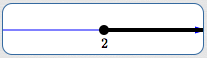union, to get the domain of $\,f\,$ $\text{dom}(f) = [-1,\infty)\,$By the way, the range of $\,f\,$ is nowhere near as easy to get from the formula.
Once you have a graph, though, the range is easy to obtain:
just use the ‘collapse the graph into the $\,y\,$-axis’ method, as described in this earlier lesson.

## Graphing a Piecewise-Defined Function

When a graph is ‘pieced together’ using different rules, as in a piecewise-defined function,
you may need up to three ‘dots’ to fully understand what's happening at a place where rules are changing.
This is illustrated in the sketch below:In this example, three rules are needed to fully understand what happens at and near $\,x\,$: There is a rule that applies at $\,x\,$. This produces the solid (filled-in) dot, with coordinates $\,\bigl(x,f(x)\bigr)\,$. This is an actual point on the graph of the function; it is an (input,output) pair. There is a rule that applies to the left of $\,x\,$. The hollow dot at $\,\color{red}{(x,\ell)}\,$ is NOT a point on the graph; it is NOT an (input,output) pair. When the input is $\,x\,$, the output is NOT $\,\ell\,$! Instead, this hollow dot is used to show limiting behavior. It answers the question: What happens to the outputs as the inputs approach $\,x\,$ from the left-hand side? (Answer: the outputs approach the number $\,\ell\,$) There is a rule that applies to the right of $\,x\,$. The hollow dot at $\,\color{green}{(x,r)}\,$ is NOT a point on the graph; it is NOT an (input,output) pair. When the input is $\,x\,$, the output is NOT $\,r\,$! Instead, this hollow dot is used to show limiting behavior. It answers the question: What happens to the outputs as the inputs approach $\,x\,$ from the right-hand side? (Answer: the outputs approach the number $\,r\,$) Remember: for a given value of $\,x\,$, there can be at most one solid dot; to be a function, each allowable input must have exactly one output.

Next, we graph: $$\cssId{s105}{f(x) = \cases { -x + 3 &\text{if }\quad -1 \le x < 1\cr 5 &\text{if }\quad 1 \le x < 2\cr x^2 &\text{if }\quad x\ge 2 }}$$ The ‘pieces’ may be graphed in any order.
Here, the choice is made to move from left-to-right on the number line (from top-to-bottom in the formula).

On $\color{red}{[-1,1)}$, $\,f\,$ is linear, since $\,\color{red}{-x+3}\,$ is of the form $\,mx + b\,$.

The endpoint $\,-1\,$ is included in the interval, indicated by the use of the bracket ‘$\,[\,$’ in interval notation.
The endpoint $\,1\,$ is excluded from the interval, indicated by the use of the parenthesis ‘$\,)\,$’ in interval notation.

$x$ is ... included or excluded? substitute into $\,-x+3\,$ plot the point ... using this kind of dot
$-1$ included $-(-1) + 3 = 1 + 3 = 4$ $(-1,4)$ solid
$1$ excluded $-(1) + 3 = -1 + 3 = 2$ $(1,2)$ hollow

Important: the point $\,(1,2)\,$ is not a point on the graph of $\,f\,$.
This hollow dot is used to show limiting behavior:
as the inputs approach $\,1\,$ from the left-hand side, the outputs approach $\,2\,$.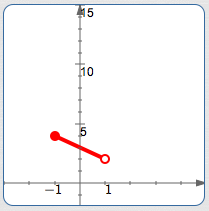On $\color{blue}{[1,2)}$, $\,f\,$ is constant with value $\color{blue}{5}$, so it graphs as a horizontal segment.

$x$ is ... included or excluded? substitute into
the constant function $5$
plot the point ... using this kind of dot
$1$ included $5$ $(1,5)$ solid
$2$ excluded $5$ $(2,5)$ hollow

Important: the point $\,(2,5)\,$ is not a point on the graph of $\,f\,$.
This hollow dot is used to show limiting behavior:
as the inputs approach $\,2\,$ from the left-hand side, the outputs approach $\,5\,$.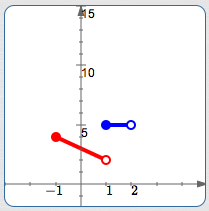On $\color{green}{[2,\infty)}$, $\,f\,$ is quadratic, since $\,\color{green}{x^2}\,$ is of the form $\,ax^2 + bx + c\,$.
Thus, it graphs as a piece of a parabola on this interval.

Think about what the entire graph of $\,y = x^2\,$ looks like (it is lightly dashed here),
and draw in the portion for the inputs $[2,\infty)\,$.

$x$ is ... included or excluded? substitute into $\,x^2$ plot the point ... using this kind of dot
$2$ included $2^2 = 4$ $(2,4)$ solidHere is the graph of the piecewise-defined function $\,f\,$, color-coded with its graph: $$\cssId{s159}{f(x) = \cases { \color{red}{-x + 3} &\text{if }\quad -1 \le x < 1\cr \color{blue}{5} &\text{if }\quad 1 \le x < 2\cr \color{green}{x^2} &\text{if }\quad x\ge 2 }}$$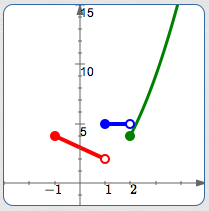## Getting the Formula for a Piecewise-Defined Function from its Graph

To go from a graph to a formula, you need additional information for any non-linear and non-constant sections, perhaps like this: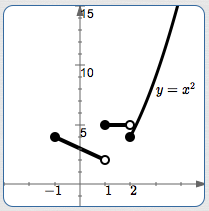There will be three rows in the piecewise-defined function, since there are three ‘pieces’.

You may want to put the proper inputs in place first;
left-to-right on the number line corresponds to top-to-bottom in the rows.
Be very careful about solid dots and hollow dots!

Put any ‘easy’ formulas in place at the same time (like constant functions and labeled pieces): $$\cssId{s169}{f(x) = \cases { ... &\text{if }\quad -1 \le x < 1\cr 5 &\text{if }\quad 1 \le x < 2\cr x^2 &\text{if }\quad x\ge 2 }}$$

It remains only to get the formula for the linear section:

• read off the coordinates $\,(-1,4)\,$ and $\,(1,2)\,$
• find the slope:   $m = \frac{2-4}{1 - (-1)} = \frac{-2}{2} = -1$
• use point-slope form with either endpoint:   $y - 2 = -(x - 1)$
• solve for $\,y\,$:   $y = -(x-1) + 2 = -x+1+2 = -x+3$
Put the formula $\,-x+3\,$ in the top row. Done!

## Variations in Notation for Piecewise-Defined Functions

There are a variety of styles for formatting piecewise-defined functions, including (but not limited to):
• using the word ‘for’ instead of ‘if’ to specify the input values
• putting commas after each rule
• putting commas at the very end of all rows but the last
Lots of different combinations are possible. Here are some examples:
 $$\cssId{s187}{ f(x) = \cases { -x + 3\color{red}{\,,} &\text{if }\quad -1 \le x < 1\cr 5\color{red}{\,,} &\text{if }\quad 1 \le x < 2\cr x^2\color{red}{\,,} &\text{if }\quad x\ge 2 }}$$ (commas after each rule) $$\cssId{s189}{f(x) = \cases { -x + 3\,, &\text{if }\quad -1 \le x < 1\color{green}{\,,}\cr 5\,, &\text{if }\quad 1 \le x < 2\color{green}{\,,}\cr x^2\,, &\text{if }\quad x\ge 2 }}$$ (commas at the very end of all rows but the last) $$\cssId{s191}{f(x) = \cases { -x + 3\,, &\text{for}\quad -1 \le x < 1\cr 5\,, &\text{for}\quad 1 \le x < 2\cr x^2\,, &\text{for}\quad x\ge 2 }}$$ (use the word ‘for’ instead of ‘if’)

With beautiful typesetting (including all the words ‘ if ’ lined up vertically), this author prefers the simplest formatting, with no extra punctuation.

Master the ideas from this section
by practicing the exercise at the bottom of this page.

When you're done practicing, move on to:
Direct and Inverse Variation

On this exercise, you will not key in your answer.
However, you can check to see if your answer is correct.
PROBLEM TYPES:
 1 2
AVAILABLE MASTERED IN PROGRESS